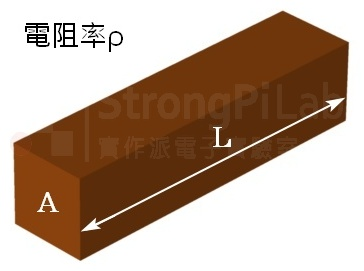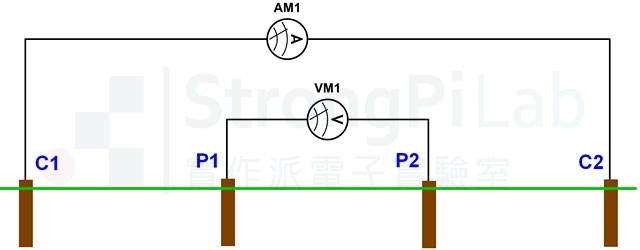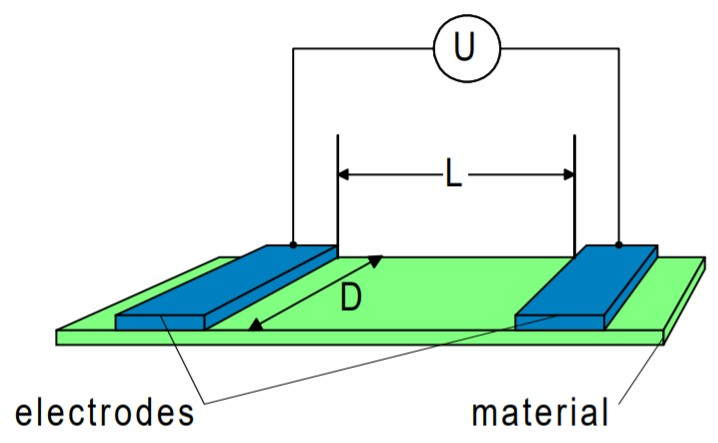# 水的電阻 是多少? 電阻率/表面電阻率 是甚麼?

## 水的電阻 Resistance 與 電阻率Resistivity電阻與電阻率的關係 $$R=\rho \frac{L}{A}$$

## 開始實驗

• 距離5cm- 1.88K ohm
• 距離10cm – 2.26K ohm
• 距離20cm – 2.66K ohm

## 計算電阻率

$$R=\rho \frac{L}{A}$$, 　$$\rho$$:電阻率, A截面積, L長度

$$R=\frac{\rho }{2\pi a}$$, 　$$\rho :電阻率$$ , a:電極的間距, R電阻Resistance measurement – 4 wires

## 表面電阻率 Surface Resistivity表面電阻率測量

## 相關文章

#### 2 Comments

1.蔡世偉表示:

請問水的電阻我用電錶的歐姆檔來測好像是不正確。請問歐姆檔為什麼會測不出來呢？
謝謝！

•阿信助教表示:

文章中測量的是水的交流電阻值, 而電錶測量的是直流電阻值, 直流電阻值會比較大, 你可以開大一點的檔位, 例如500k ohm檔位來測量看看